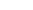A   B   C   D   E   F   G   H   I   J   K   L   M   N   O   P   Q   R   S   T   U   V   W   X   Y   Z

File Transfer Protocol (FTP): A network protocol that allows computers to send and receive files over IP networks, including the Internet.

Floating Point Arithmetic: Binary arithmetic that approximates decimal numbers, including negative numbers and numbers between 0 and 1, within a fixed number of bits. For example, in a 32-bit "word" the first bit indicates whether the number is positive or negative and the next eight bits determine where the decimal point lies in the number represented by the remaining 23 bits. Floating point arithmetic is the basis of numerical computation.

FTP: See File Transfer Protocol.

Fuzzy Logic: A system of logic that includes the concept of partial truth in order to accommodate uncertainty and imprecision.

A   B   C   D   E   F   G   H   I   J   K   L   M   N   O   P   Q   R   S   T   U   V   W   X   Y   Z